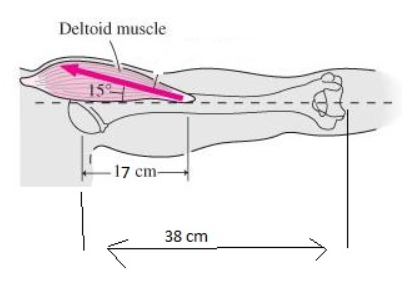# Problem: If you hold your arm outstretched with palm upward, as in the figure, the force to keep your arm from falling comes from your deltoid muscle. The arm of a typical person has a mass of 4.0kg and the distances and angles are shown in the figure. a. What force must the deltoid muscle provide to keep the arm in this position? b. By what factor does the force exceed the weight of the arm?

###### FREE Expert Solution

Torque;

$\overline{){\mathbit{\tau }}{\mathbf{=}}{\mathbit{r}}{\mathbit{F}}{\mathbf{·}}{\mathbf{sin}}{\mathbit{\theta }}}$

(a)

The weight of the arm creates a clockwise torque, while the Deltoid muscle creates a counter-clockwise torque.

Tcw = r•mg sinθ = 0.38 × 4.0 × 9.8 × sin 90

Tccw = r•FD sinθ = 0.17 × FD × sin 15

98% (263 ratings)###### Problem Details

If you hold your arm outstretched with palm upward, as in the figure, the force to keep your arm from falling comes from your deltoid muscle. The arm of a typical person has a mass of 4.0kg and the distances and angles are shown in the figure.

a. What force must the deltoid muscle provide to keep the arm in this position?

b. By what factor does the force exceed the weight of the arm?